# NeuroGeometry - Analysing 3D morphology of neurons

## Introduction

One of the key distinguishing features of nat is the ability to analyse neuronal morphology and connectivity from a geometric perspective. In other words nat provides functions to analyse the placement of neurons within the context of the brain and the organisation of local circuits.
This vignette will give a few examples, but this is only skimming the surface of what is possible.

If you see an analysis in a paper associated with the Jefferis lab but cannot figure out how to use nat to achieve this, then feel free to contact the nat–user Google group to ask for help.

## Vertex information

library(nat)

## Loading required package: rgl

## Registered S3 method overwritten by 'nat':
##   method             from
##   as.mesh3d.ashape3d rgl

##
## Attaching package: 'nat'

## The following objects are masked from 'package:base':
##
##     intersect, setdiff, union


One of the key tools for geometric work in nat is the xyzmatrix function, which extracts 3-D coordinate information from any object containing vertices.

n=Cell07PNs[]
xyz=xyzmatrix(n)
summary(xyz)

##        X               Y                Z
##  Min.   :186.9   Min.   : 90.36   Min.   : 88.2
##  1st Qu.:225.6   1st Qu.: 97.56   1st Qu.:103.4
##  Median :258.4   Median :102.70   Median :112.9
##  Mean   :249.4   Mean   :104.03   Mean   :120.9
##  3rd Qu.:277.7   3rd Qu.:109.04   3rd Qu.:139.0
##  Max.   :289.5   Max.   :132.71   Max.   :157.3

plot(xyz[,c("X","Y")], type='p')This also works for neuronlists, so we can extract all of the points in a collection of neurons:

xyz=xyzmatrix(Cell07PNs)


We could ask for all the points close to a specific X coordinate

close_to_x250=abs(xyz[,'X']-250)<10
table(close_to_x250)

## close_to_x250
## FALSE  TRUE
## 20479  1728


We can also select points interactively by using the rgl::select3d function.

## Worked example - plane cutting a tract

As a worked example let’s try to find the plane cutting a tract defined by the axons of some example neurons:

plot(Cell07PNs, WithNodes=F)
dist=10
abline(v=250, col='red')
abline(v=c(250-dist, 250+dist), col='red', lty='dotted')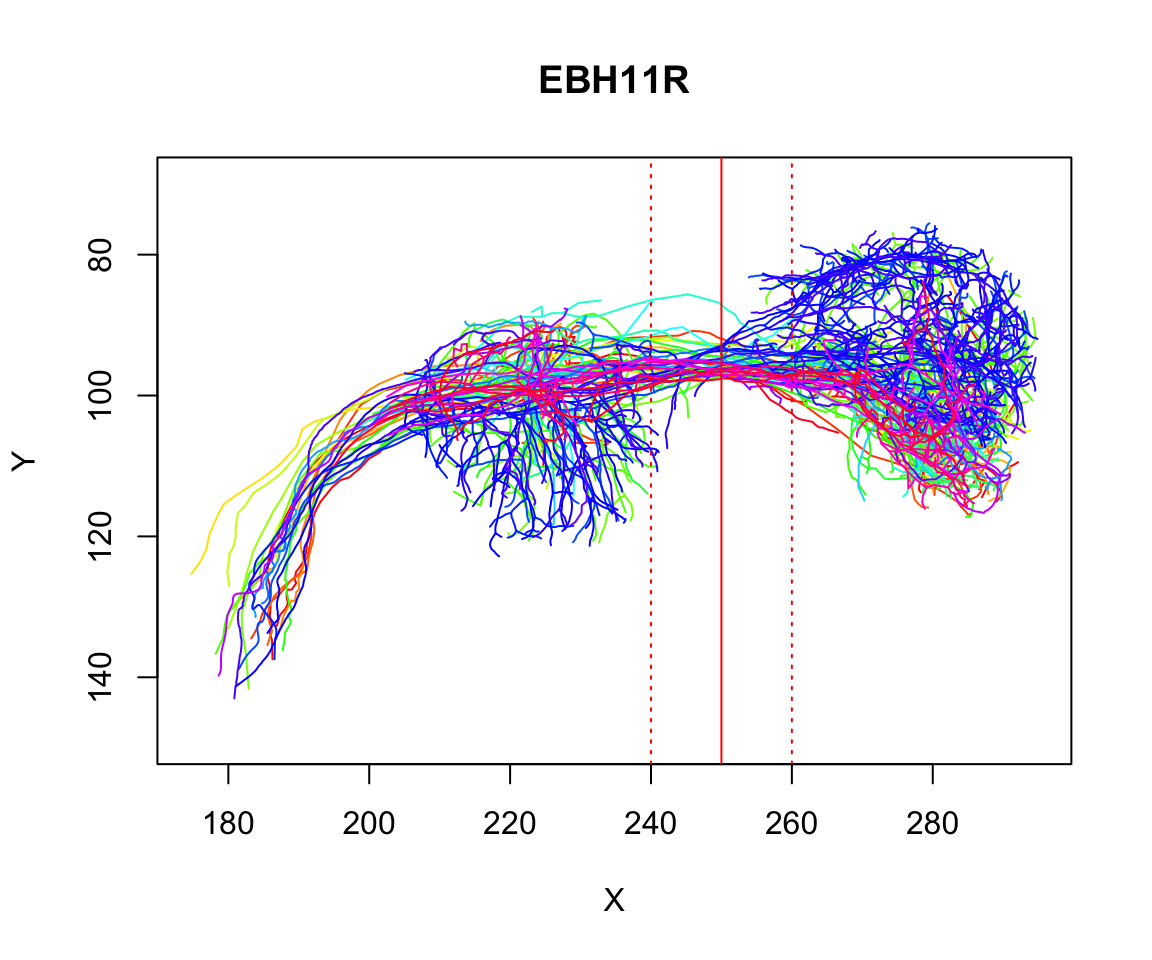We could ask for all the points close to a specific X coordinate

close_to_x250=abs(xyz[,'X']-250)<10
table(close_to_x250)

## close_to_x250
## FALSE  TRUE
## 20479  1728


Let’s find the centroid of those selected points:

centroid=colMeans(xyz[close_to_x250,])
centroid

##         X         Y         Z
## 251.16788  94.76735 141.11495

plot(Cell07PNs, WithNodes=F)
points(matrix(centroid[1:2],ncol=2), pch=20)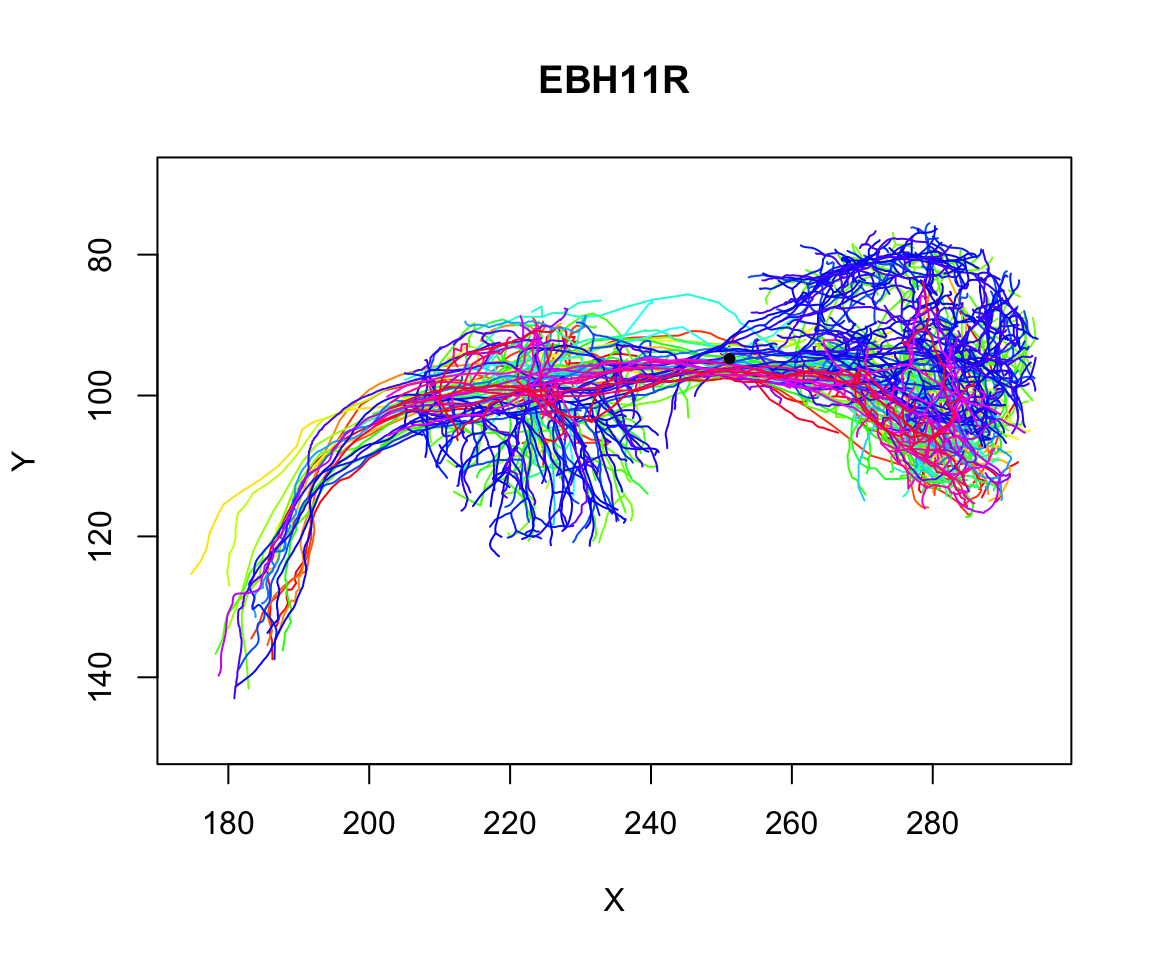### PCA to find tract vector

Now let’s do a principal components analysis on those points

pc=prcomp(xyz[close_to_x250,])
pc1=pc$rotation[,'PC1'] plot(Cell07PNs, WithNodes=F) points(matrix(centroid[1:2],ncol=2), pch=20) res=rbind(centroid-pc1*10, centroid, centroid+pc1*10) lines(res[,1:2])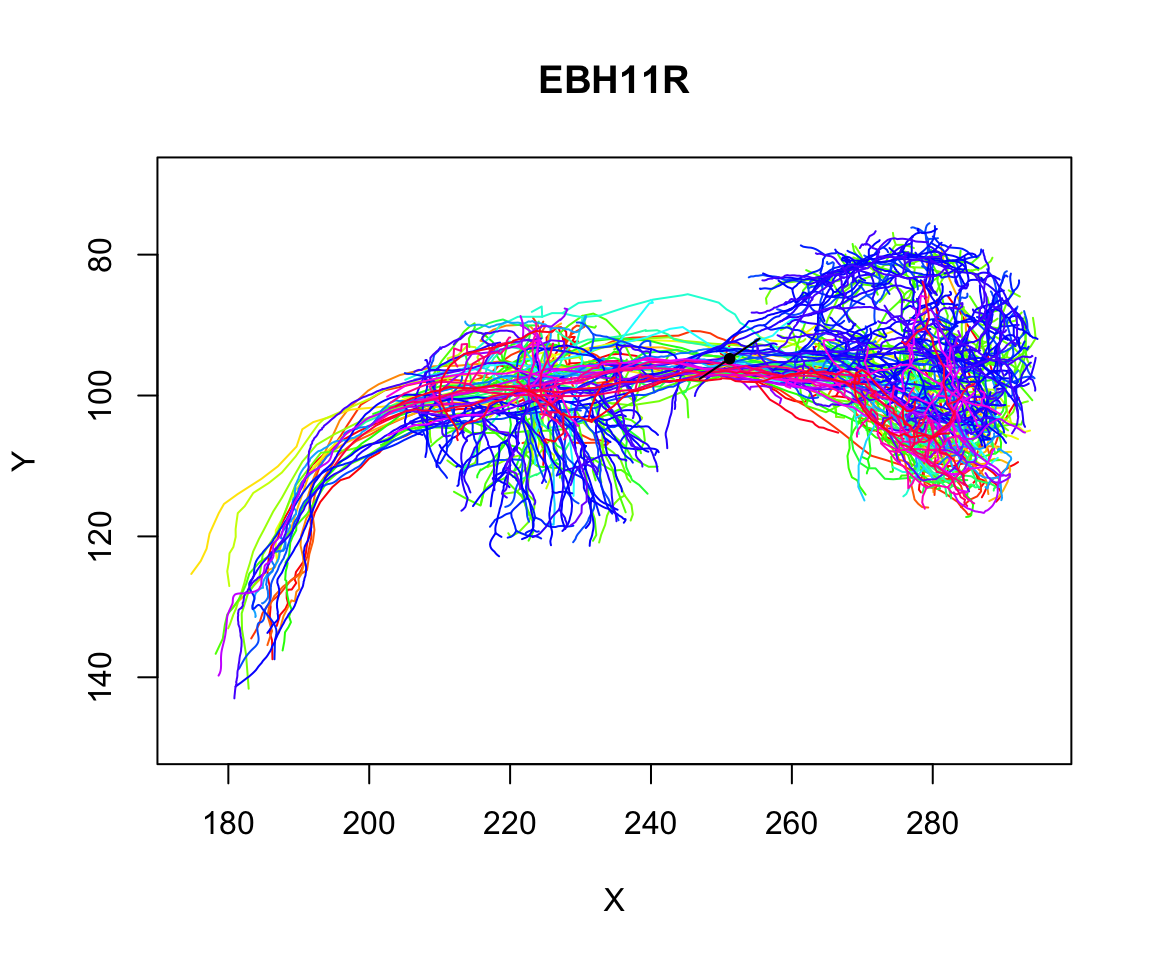OK, perhaps it would be better if we only used the spine of the neurons # There is a problem with one neuron with a loop, hence OmitFailures spines=nlapply(Cell07PNs, spine, UseStartPoint=T, OmitFailures = T)  ## Warning in get.shortest.paths(ng, from = from, to = to, mode = "all"): At ## structural_properties.c:4597 :Couldn't reach some vertices  xyz=xyzmatrix(spines) close_to_x250=abs(xyz[,'X']-250)<10 table(close_to_x250)  ## close_to_x250 ## FALSE TRUE ## 5137 876  pc=prcomp(xyz[close_to_x250,]) pc1=pc$rotation[,'PC1']
plot(spines, WithNodes=F)
points(matrix(centroid[1:2],ncol=2), pch=20)
res=rbind(centroid-pc1*10, centroid, centroid+pc1*10)
# nb 1:2 => just the xy coords
lines(res[,1:2])### Tract vector for each neuron

Alternatively, we could find the vector of the first PC for each neuron. Let’s write a function to do that and then apply it to the spine of each neuron.

# returns 6 vector
# first 3 values are centroid
# second three values first principal component
tract_vector <- function(n, xval=250, thresh=10) {
p=xyzmatrix(n)
near=abs(p[,'X']-xval)<thresh
pc=prcomp(p[near,])
c(colMeans(p[near,]), pc$rotation[,'PC1']) } tvs=sapply(spines, tract_vector) mean_cent=rowMeans(tvs[1:3,]) mean_vec=rowMeans(tvs[4:6,]) # nb *10 to make the vector longer (10 µm) and more visible res2=rbind(mean_cent-mean_vec*10, mean_cent, mean_cent+mean_vec*10) plot(spines, col='grey', WithNodes=F) lines(res2[,1:2], lwd=3)### Lines crossing a plane We can look at the plane we have defined in a 3D context as follows: plc=plane_coefficients(mean_cent, mean_vec) plot3d(Cell07PNs[c(1,30)], col='grey', WithNodes = F) planes3d(plc[,1:3], d=plc[,'d'])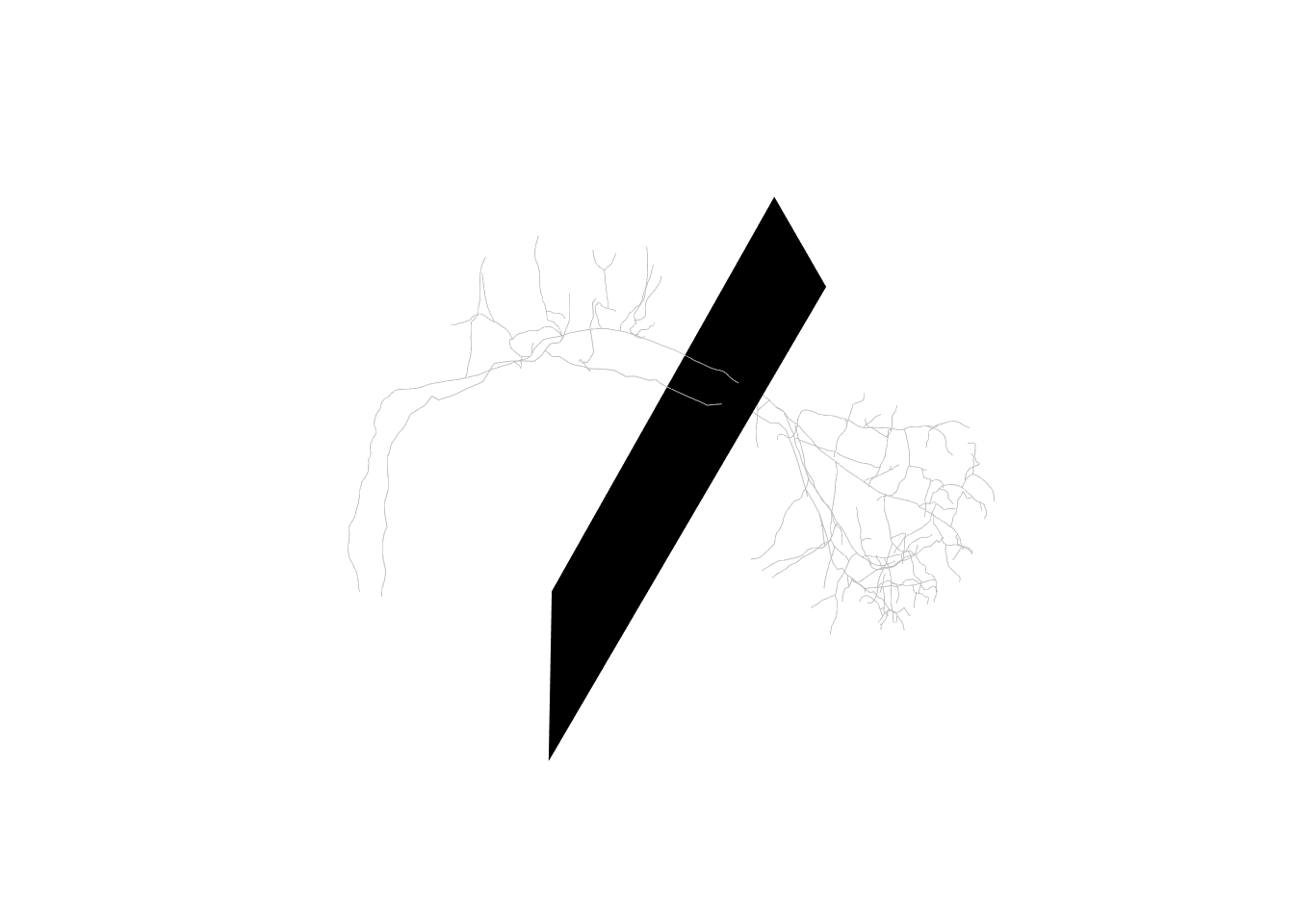We can also make a small square plane centred on the chosen point library(rgl) clear3d() make_centred_plane <- function(point, normal, scale = 1) { # find the two orthogonal vectors to this one uv = Morpho::tangentPlane(normal) uv = sapply(uv, "*", scale, simplify = F) qmesh3d( cbind( point + uv$z,
point + uv$y, point - uv$z,
point - uv\$y
),
homogeneous = F,
indices = 1:4
)
}
plane=make_centred_plane(mean_cent, mean_vec, scale=15)
plot3d(spines, col='grey')
shade3d(plane, col='red')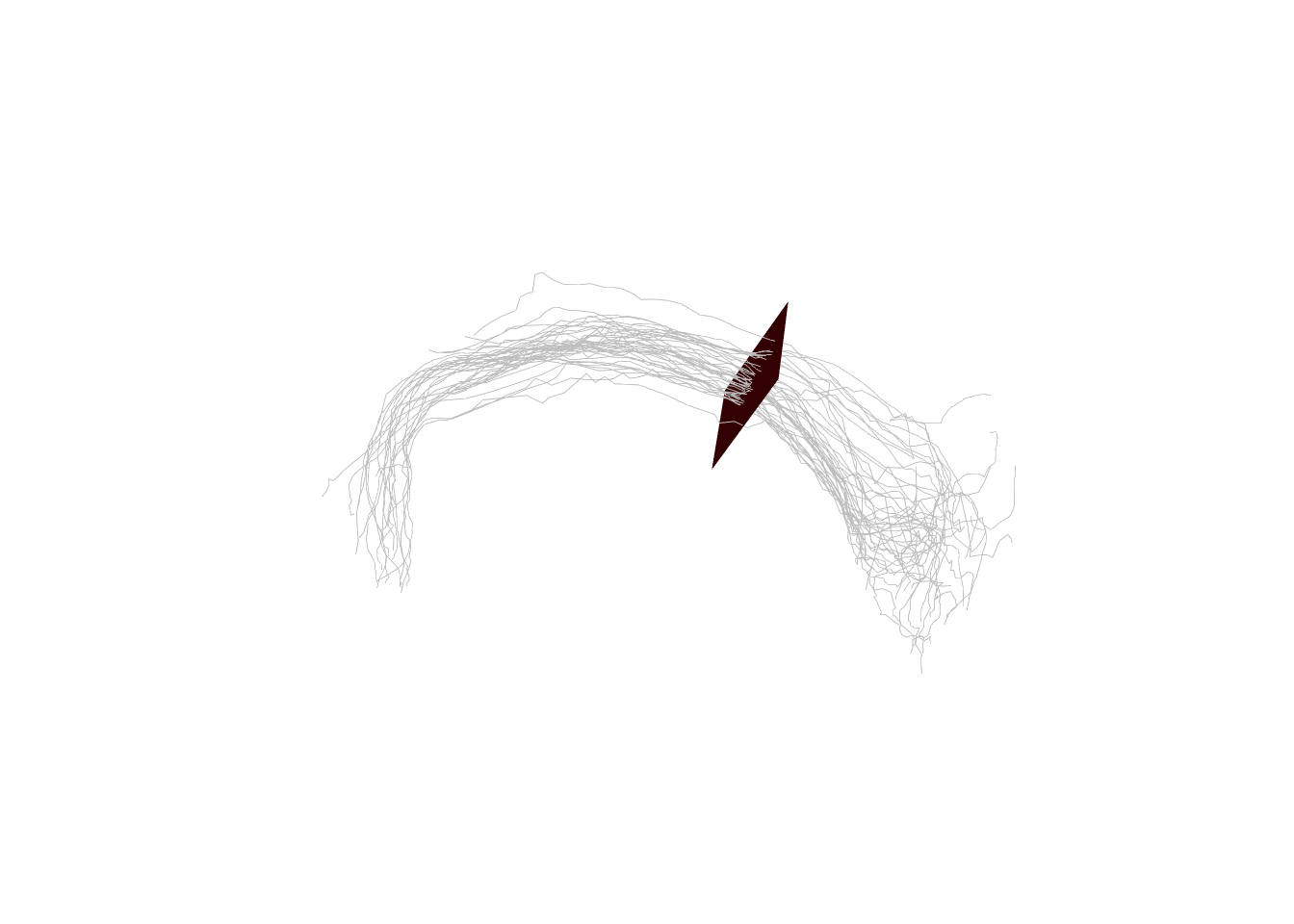Finally, we can find the positions at which each neuron intersects the plane. Note that when there are multiple intersections, we only choose the closest point to the centroid that we calculated when defining the plane.

intersections=t(sapply(Cell07PNs, intersect_plane, plc, closestpoint=mean_cent))
# center those intersection points
intersections.cent=scale(intersections, center = T, scale = F)
d=sqrt(rowSums(intersections.cent^2))
mean(d)

##  3.118692


Then we can plot those positions on the plane

spheres3d(intersections, col='red', rad=.5)
shade3d(plane, col='black')
plot3d(spines, col='grey')


You must enable Javascript to view this page properly.

Finally we can construct a 2D coordinate system on the plane and project the intersection positions onto that.

# find pair of orthogonal tangent vectors of plane
uv = Morpho::tangentPlane(mean_vec)
# centre our points on mean
sp=scale(intersections, scale = F, center=T)
DotProduct=function(a,b) sum(a*b)
# find coordinates w.r.t. to our two basis vectors
xy=data.frame(u=apply(sp, 1, DotProduct, uv[]),
v=apply(sp, 1, DotProduct, uv[]))
# maintain consist x-y aspect ratio
plot(xy, pch=19, asp=1, main = "Position of Axon intersection in plane",
xlab="u /µm", ylab="v /µm")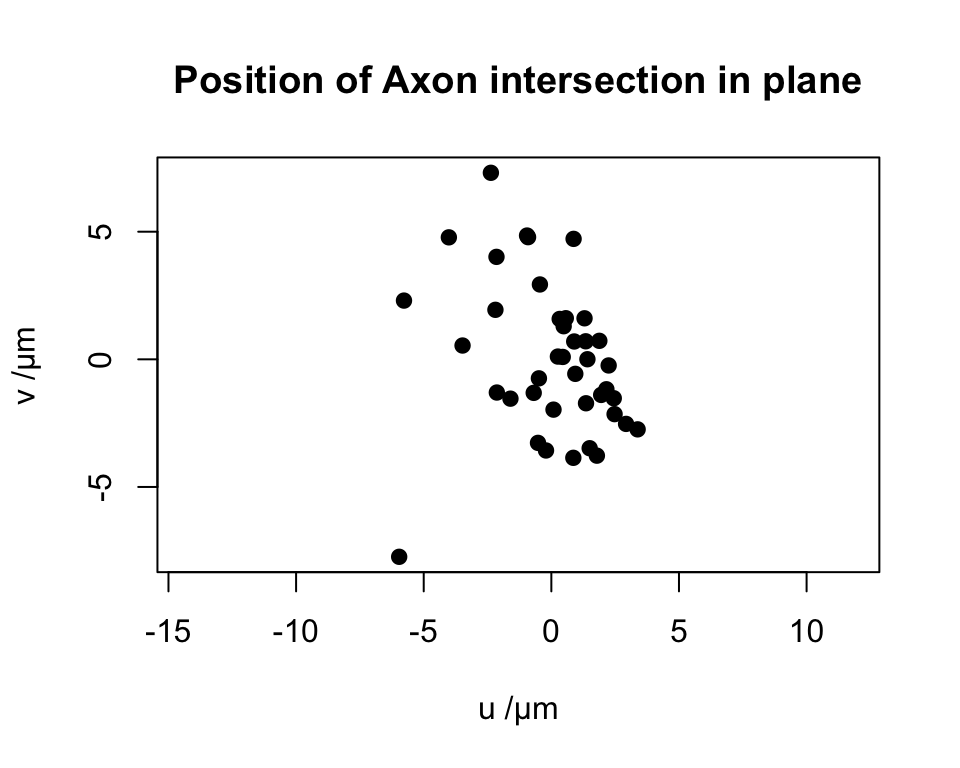By: Gregory Jefferis
Contents